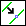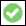> > Split an edge and introduce a vertex

Split an edge and introduce a vertex
1. Click Modeling and then, in the Modify 3D group, click More.
2. ClickSplit Edge in the Tools section. The Split Edge dialog box opens.
3. Click the edge or 3D curve to split.
4. Either click a split point on the edge (creating a vertex at that point), or select one of the following options and split the edge as described:
Midpoint: a vertex is generated at the midpoint of the edge or 3D curve, creating two equal segments.
Segments: Type a positive number that specifies how many segments of equal length are created. You can also type non-integral numbers. The decimal produces a smaller segment proportional in length to one of the "whole" segments by that fractional value. For example, splitting an edge into 2.5 segments produces two equidistant segments and one segment half of length.
Length: Type a positive number that specifies the split point measured from the begin point of the edge. Type a negative number that specifies the split point measured from the end point of the edge. The begin and end points are labeled with their distances when you select this option.
Elem 3D: Select a 3D element (edge, face, or workplane) in the viewport. The selected edge splits where it intersects with the 3D element. If more than one intersection point exists, the point closest to the spot where you selected the edge is used.
5. Clickto complete the operation.